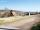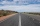Kite

John a kite, which is diamond shaped. Its diagonals are 60 cm long and 90 cm long. Calculate:

a) the diamond side
b) how much paper John needs to make a kite if he needs paper on both sides and needs 5% of the paper for bending.

Result

a =  54.083 cm
b =  5670 cm2

Solution:Leave us a comment of example and its solution (i.e. if it is still somewhat unclear...):Be the first to comment!To solve this example are needed these knowledge from mathematics:

Our percentage calculator will help you quickly calculate various typical tasks with percentages. Pythagorean theorem is the base for the right triangle calculator.

Next similar examples:

1. AcreagePlot has a diamond shape, its side is 25.6 m long and the distance of the opposite sides is 22.2 meters. Calculate its acreage.
2. Diagonals in diamons/rhombusRhombus ABCD has side length AB = 4 cm and a length of one diagonal of 6.4 cm. Calculate the length of the other diagonal.
3. TVsProduction of television sets increased from 3,500 units to 4,200 units. Calculate the percentage of production increase.
4. SatinSanusha buys a piece of satin 2.4 m wide. The diagonal length of the fabric is 4m. What is the length of the piece of satin?
5. Horizontal distanceThe road has a gradient of 8%. How many meters will the road rise on a horizontal distance of 400m?
6. ClassIn 7.C clss are 10 girls and 20 boys. Yesterday was missing 20% of girls and 50% boys. What percentage of students missing?
7. Seeds 2How many seeds germinated from 1000 pcs, when 23% no emergence?
8. PercentsHow many percents is 900 greater than the number 750?
9. Apples 2James has 13 apples. He has 30 percent more apples than Sam. How many apples has Sam?
10. Percents - easyHow many percent is 432 out of 434?
11. PersonsPersons surveyed:100 with result: Volleyball=15% Baseball=9% Sepak Takraw=8% Pingpong=8% Basketball=60% Find the average how many like Basketball and Volleyball. Please show your solution.
12. The percentages in practiceIf every tenth apple on the tree is rotten it can be expressed by percentages: 10% of the apples on the tree is rotten. Tell percent using the following information: a. in June rained 6 days b, increase worker pay 500 euros to 50 euros c, grabbed 21 fro
13. Broken treeThe tree was 35 meters high. The tree broke at a height of 10 m above the ground. Top but does not fall off it refuted on the ground. How far from the base of the tree lay its peak?
14. Highway repairThe highway repair was planned for 15 days. However, it was reduced by 30%. How many days did the repair of the highway last?
15. New refrigeratorNew refrigerator sells for 1024 USD, Monday will be 25% discount. How much USD will save, and what will be the price?
16. EnrollmentThe enrollment at a local college increased 4% over last year's enrollment of 8548. Find the increase in enrollment (x1) and the current enrollment (x2).
17. CancerOf the 80 people 50 people ill cancer. What percentage of people isn't ill?